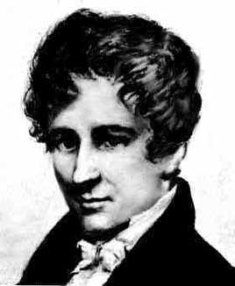# AbelAllPages RecentChanges Links to this page Edit this page Search Entry portal Advice For New UsersNiels Henrik Abel 1802 - 1829

Norwegian mathematician who proved that it is impossible to find a formula to solve fifth degree (quintic) polynomial equations using radicals.

This doesn't mean that quintic equations don't have solutions or that the solutions cannot be found. The fundamental theorem of algebra states that an nth degree polynomial has n solutions and there are iterative methods to find solutions of polynomial equations to any accuracy.

Abel showed that it is impossible to find the solution of all quintic (and higher order) polynomial equations using a finite number of operations of the form addition, subtraction, multiplication, division and root extraction acting on the coefficients of the polynomial.

One of the famous people on this site